Impact Factor 2.705 | CiteScore 2.2
More on impact ›

# Frontiers in Materials## Original Research ARTICLE

Front. Mater., 10 April 2019 | https://doi.org/10.3389/fmats.2019.00056

# Rheological Properties of Polyurethane-Based Magnetorheological GelsGuang Zhang1,Yancheng Li2,3*,Huixing Wang1 andJiong Wang1*
• 1School of Mechanical Engineering, Nanjing University of Science and Technology, Nanjing, China
• 2College of Civil Engineering, Nanjing Tech University, Nanjing, China
• 3School of Civil and Environmental Engineering, University of Technology Sydney, Ultimo, NSW, Australia

The paper tests the influence of mass fractions of carbonyl iron particles (CIPs) on the rheological properties of magnetorheological (MR) gels. Polyurethane-based MR gels with different weight fraction of CIPs, i.e., 40, 60, and 80%, were firstly prepared by mechanical mixing, respectively. The changes of shear stress and viscosity with shear rate under different magnetic flux density were tested and analyzed. It was found that the shear stress increases with mass fraction under magnetic flux density. The viscoelastic properties of MRGs were achieved by oscillatory shear measure. The effects of strain amplitude and frequency on viscoelastic of MRGs under different magnetic flux density were measured and analyzed. The study results shown that the elastic characteristics become more obvious with the increase of CIPs mass fraction. However, it has opposite effect on the viscous properties of materials.

## Introduction

MRG is a new generation of MR smart materials with soft magnetic particles uniformly suspending in non-magnetic carriers. Fast and reversible magnetorheological effect will occur under the applied of magnetic field (Gan et al., 2017). It was more than two decades when Shiga et al. first reported silicone-based MR gel for solving the problem of particle settling in 1995 by dispersing the CIPs into silicone polymer colloid uniformly and since then MR gel has been considered as a momentous member of MR intelligent materials (Shiga et al., 1995). MR gels bring out larger MR effect than MR elastomer while overcoming the sedimentation issue associated with MR fluids, which can be regarded as an intermediate product between MR fluid and MR elastomer (Wilson et al., 2002; Wei et al., 2010; Zhang et al., 2010; Xu et al., 2013; Miao et al., 2014). MR gel is normally composed of micron or submicron soft magnetic particles (such as CIPs and pure iron powders), crosslink polymer liquid (such silicone resin and polyurethane), and additives (Ju et al., 2013). Notably, the MR gel shows a colloidal with a certain shear yield stress without magnetic field due to its matrix. Once the magnetic field is applied, the MR gel will transform into a solid state in a very short time (within milliseconds) and will exhibit a higher degree of shear yield stress. This process has a continuous and reversible characteristic (Hu et al., 2010; An et al., 2012). The above characteristics indicate that MR gel carries the basic rheological properties of the MR material and has larger magnetorheological effect and better settlement resistance, which offers a reliable and simple solution to design brakes (Wilson et al., 2002), dampers (Yang et al., 2015), and isolators (Xu et al., 2013). However, the research on MR gel-like material is still in its early stage and much work are to be conducted. The researchers in the field were initially from chemistry with focus on heat flow and conversion of the polymeric matrix, and it is now gradually shifting to the stage with more engineers involved. From an application point of view, the mechanical properties of the material under influence of magnetic field, such as shear yield stress, viscosity, storage modulus, loss factor and MR effect, are of great interest and hence should be carefully investigated.

Owing to its great potentials, understanding its rheological properties holds the key to unlock reliable and efficient usage in engineering applications. Among the main composition, the soft magnetic particles play the key role to activate the MR effect and hence their impact should be carefully investigated. The influences of CIP contents on magneto-mechanical performances of MR materials have been tested by different researchers. Fuchs et al. prepared two silicone-based MR gel samples with different CIP contents. Variable magneto-induced features were examined by rheometer sweeping of shear rate from 20 to 6,000 s−1. It was found that the silicone-based MR gel with 84.5 wt% CIPs has a stable shear yield stress over a wide range of shear rate (Fuchs et al., 2004). Xu et al. prepared several MR gels consisting plastic polyurethane matrix swollen by non-volatile solvent in different weight fractions of CIPs and their MR properties (both shear yield stress and modulus) were tested and analyzed. It was shown that the carrier has little effect on the linear viscoelastic (LVE) region of MRGs, though the magnitude the storage modulus G' can be greatly changed by the solvent content (Xu et al., 2013). The dynamic magneto-induced properties of the shear-stiffening gel under compression was measured by Wang et al. It was found that CIP contents have great influences on dynamic properties (Wang et al., 2016). Xu et al. developed MR gels with different weight fractions and the stress evolution characteristics of MRGs under stepwise shear loading are tested. It was found that the mass fraction of CIPs has a significant effect on the rheological properties of prepared materials. The dynamic modulus and the plain modulus increase along with CIPs weight fraction (Xu et al., 2017). These results indicate that the CIP weight fraction has a significant impact on the magneto-mechanical features of MR materials.

In addition, the basic carrier has a great impact on the rheological properties of MR materials (Xu et al., 2011). Mitsumata et al. firstly prepared carrageenan/iron oxide MR gels and the reversible rheological properties were tested. It was found that great variety of the storage modulus was almost not exhibited when the carrageenan concentration was 2 wt%; however, the MR effect appeared on reduced concentration. The limit variation in the relative storage modulus was 24 when the carrageenan concentration was 0.6 wt% (Mitsumata and Abe, 2011). Zhang et al. developed silicone-based MR gels by with 30, 50, and 70 wt% CIPs and the rheological properties were systematically tested, respectively. It was found that the content of silicone has a great influence on the zero-field viscosity, shear stress and response rate, and MR gel with 30 wt% silicone has the best MR effect (Zhang et al., 2018). Wilson et al. self-developed series of PUs and silicones matrix by controlling the weight of reactants and the content of modifier (reacting or non-reacting) and then developed PU-based and silicone-based MR gels, respectively. It was found that rheological property can be qualitatively controlled of as-developed MR products by adjusting the ratio of reactants and diluents. The PU and silicone polymers have three existing states, that is solid, gel, and liquid forms, according to the crosslinking and dilution (Wilson et al., 2002). Xu et al. developed a novel high-performance PU-based MR material. The dynamic properties of the MR material were systematically measured and the influences of the PU contents were analyzed. It was found that the material with 20% PU matrix weight fraction shows a high dynamic property (Xu et al., 2011). Fuchs et al. developed polyurethane (PU)-based and silicone-based MR gels, respectively. In this work, tunable rheological properties and settling rate were investigated. The results show that PU-based has the better particle settling resistance and has a high shear yield stress (23 kPa at 350 mT). Both dynamic and static shear stress values of the samples were found to be similar in magnitude: the dynamic and static shear stress of both PU and silicone-based produces with 84.5% weight mass CIPs under 120 mT range from 5 to 8 kPa (Fuchs et al., 2004). PU have attracted the attention of researchers among the various polymer matrixes due to better degradation stability than natural rubber and superior mechanical properties compared with silicone rubber. Interestingly, it is found that PU can be tuned from flow-able liquid to semi solid (gel like) depending upon the degree of crosslinking (Mordina et al., 2016). Accordingly, an appropriate carrier should be selected to prepare stable MR gels for obtaining a suitable modulus and wider magnetorheological effect. In addition, MRG based on PU has better sedimentation resistance and particle aggregation resistance (Wilson et al., 2002; Yang et al., 2015). The above characteristics can greatly simplify the sealing structure in the device structure design with MRG as the medium, thus reducing the cost, and is of certain significance to improve the stability of the device.

To this point, this research is to investigate the influence of the CIP fractions on their rheological properties with commercially available PU matrix. PU-based MR gels with CIP mass fractions of 40, 60, and 80% are freshly developed and the magnetic properties and microstructure were tested and analyzed accordingly. Then the magneto-mechanical features of MR gel under continuous shear with different CIP weight fractions are measured and discussed. Finally, the viscoelastic properties of MRGs were achieved by oscillatory shear measure. The effects of magnetic flux density, shear rate, strain amplitude and driving frequency on the dynamic properties of MR gel with different CIP weight fractions were discussed comprehensively.

## MR Gels Preparation

In this study, the synthesis of the MR gels was carried out by mixing CIPs (type: JCF2-2, JiLin Jien Nickel Industry Co., Ltd, China, with an average particle size of about 5 μm) into a commercial PU (Shanghai mile Chemical Technology Co., Ltd, China). The several important physical properties of PU employed in the study are exhibited in Table 1. The CIPs mass fraction of MR gels are selected as 40, 60, and 80%, respectively.

TABLE 1

There are three-prongs in the development of MR gels. Firstly, weighing the exact quantity of the PU and CIPs. Placing PU matrix into three 250 ml beakers. Secondly, the carrier (PU) was mechanically stirred at 400 rpm for 30 min in the environment of a constant temperature (65°C) in order to make the CIPs dispersed more uniformly. Finally, the CIPs with 40, 60, and 80% weight fractions were added into the accommodating matrix and were stirred at 750 rmp until the PU matrix and CIPs were fully and evenly mixed. In this paper, the three MRG samples were named as MRG-40, MRG-60, and MRG-80, respectively.

## Properties Characterization

The magnetic hysteresis characteristic of CIP and MR gels with CIPs weight fraction of 40, 60, and 80 % were measured by using the vibrating sample magnetometer (VSM, Lake Shore 7407, USA) at the temperature of 25°C. A commercial rheometer (Physica MCR 302, Anton Paar Co., Austria) with plate (the diameter is 20 mm) was employed to measure the magnetic-induced mechanical properties of MR gels by continuous shear and oscillatory shear tests. The magnetic field generated by the coil can be controlled by regulating the current through the coil. The gap between the plate and the base was maintained at 1 mm so as to emerge symmetrical magnetic flux density range from 0 to 1,200 mT through the samples by adjusting the current in the coil from 0 to 5 A.

Rotational and oscillatory shear test are the most frequently used testing methods to investigate the rheological properties of materials. Scanning test of magnetic flux density (changing from 0 to 1,200 mT) was carried out under a constant shear rate of 50 s−1 during shear rotation test. The shear stress and viscosity of the products were tested under different magnetic flux densities (0, 240, 480, 720, and 960 mT), with shear rate from 1 to 100 s−1. The dynamic properties of samples were captured by mean of oscillation shear. The amplitude changes from 0.01 to 10% under the strain amplitude sweeping at a constant frequency of 5 Hz. The oscillation frequency changes from 5 to 100 Hz for scanning of frequency measurements at a constant strain amplitude of 0.01%. Both the scanning measurements of strain amplitude and frequency were carried out under the four magnetic flux densities, which are 240, 480, 720, and 960 mT. In order to ensure the comparability of the test data, the pre-shearing process with a shear rate of 50 s−1 for 3 min was carried out before each step was measured.

## Results and Discussion

### Magnetic Properties

The magnetic hysteresis properties of pure CIP and MR gels with different CIP content, i.e., 40, 60, and 80 wt%, were investigated by VSM. Figure 1 display the magnetic properties of as-developed MR gels with various weight fraction of CIPs, which indicated that the samples exhibits obvious soft magnetic property. The moment increases linearly with the increase of magnetic field and then goes to saturation value. For CIP, the yielding starts around 5,000 Oe and when the external magnetic field strength increases to 8,000 Oe, CIP reaches a magnetic saturation state with a saturation moment of 206 emu/g. For MR gels, the CIPs weight fraction has great influence on saturation moment. MR gel with higher CIP weight fraction have higher saturated moment, i.e., about 54 emu/g for MRG-40, 91 emu/g for MRG-60, and 107 emu/g for MRG-80.

FIGURE 1

### Magnetic Field Sweep

The magnetic flux density dependence of shear stress for different samples in small strain (linear viscoelastic range) was shown in Figure 2. The magnetic flux density has a significant influence on the mechanical properties of samples. Shear stress increases with the enhancement of the magnetic flux density under the constant shear rate. When the magnetic flux density is very small, i.e., B < 100 mT, the shear stress of the samples changes slightly. With the increase of the magnetic flux density, the shear stress of the materials changes approximately linear at moderate magnetic field. When the magnetic flux densities are 700 and 900 mT, the shear stresses of MRG-40 and MRG-60 reach saturation value, 8 and 20 kPa, respectively; however, when the magnetic flux density increases to 1,200 mT, the shear stress of MRG-80 does not reach the saturation value, but its growth rate decreases obviously. Therefore, the change is roughly divided into three kinds of growth: the initial growth (from Figures 3A,B), the linear growth (from Figures 3B,C), and the saturate tendency (from Figures 3C,D). The change of magnetic-induced micro-structure of samples can be interpolated as the process that the CIPs inside material attempt to overcome the internal friction resistance (mainly the resistance of the polymer matrix). At the initial stage, the intertwined polymer chains are flexible along the moving direction under the drive of particles, and the damping force to restrict particles motion weakens slightly. Therefore, the chain forming process is also slow, and the change of microstructure is as shown from Figures 3A,B. With the increase of magnetic flux density, the particle chain presents a linear growth process in the flexible polymer chain environment as shown from Figures 3B,C. Further enhancing the magnetic field, the free particles combine into chains and become scarce as shown from Figures 3C,D, which leads to the saturated shear stress with the increasing of magnetic flux density. This phenomenon coincide with the hysteresis curve of samples in Figure 1.

FIGURE 2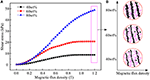Figure 2. (A) Shear stress as a function of magnetic flux density at $\stackrel{·}{\gamma }$ = 50 s−1for samples; (B) microscopic chain structure (red solid line represents polyurethane polymer, and solid small circle represents CIPs).

FIGURE 3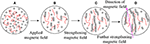Figure 3. Internal chain forming process (red solid line represents polyurethane polymer, and hollow small circle represents CIPs). (A) no magnetic field; (B) applying a weak magnetic field; (C) magnetic field enhancement and (D) further strengthening of magnetic field.

### Rheology Properties in Rotational Shear

The shear stress and viscosity curves along shear rate of MR gels with various CIP weight fractions under different magnetic flux densities are exhibited in Figures 4, 5, respectively. As shown in Figure 4A, in the absence of an external magnetic field, the shear stress increases linearly along with shear rate, which shows Newtonian fluid characteristics. With the influence of the magnetic flux density, the MR gel behaves as non-Newtonian fluid which needs to overcome a certain amount of the stress (named as yield stress) to become fluidic state, i.e., Bingham plastic behavior. It is shown that the yield stress increases with weight fraction at a given magnetic flux density. The magnetic flux density has a great impact on the yield stress of prepared MR materials, which changes from 2 to 10 kPa for 40 wt% MR gel and from 5.6 to 45 kPa for 80 wt% MR gel sample. This is due to the stronger interaction in chain-like structure at higher magnetic flux density level. After the yield stress, the shear stress has a slight increase along shear rate for the magnetic flux density below saturated magnetic flux density, which should be below 960 mT as under such conditions the MR gel has distinct saturation yield stress. It implies that the better damping property at lower magnetic flux density levels. Under the magnetic flux density of 960 mT, the MR gel presents a slight shear thinning behavior, especially for 80 wt% sample, which has slight decreasing shear stress against shear rate.

FIGURE 4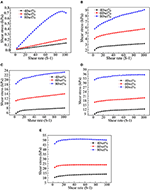Figure 4. Shear stress vs. shear rate under different magnetic flux densities (A: B = 0 mT; B: B = 240 mT; C: B = 480 mT; D: B = 720 mT; E: B = 960 mT).

FIGURE 5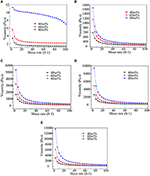Figure 5. Viscosity vs. shear rate under different magnetic flux densities (A: B = 0 mT; B: B = 240 mT; C: B = 480 mT; D: B = 720 mT; E: B = 960 mT).

The viscosity of the MR gel decreases along shear rate at low shear rate level, i.e., below 20 s−1, shown in Figure 5. Such behavior is more distinct under the influence of the magnetic flux density. The CIP content has considerable impact on the viscosity of the MR gel with and without magnetic flux density. For example, at the magnetic flux density of 960 mT, the MRG-80 has a viscosity of 503 Pa.s at the shear rate of 100 s−1; with lower fraction of CIPs as 40 wt% the viscosity of the sample has significant reduction to 139 Pa.s.

### Rheological Properties Under Oscillatory Shear

Storage modulus and loss modulus are the key mechanical parameters used to reveal the dynamic properties of materials (Xu et al., 2013). Among them, the storage modulus is an important parameter to characterize the elastic properties of materials, which can show the ability of materials to store energy (have the feature that all the energy completely available after the external load is removed) after shear deformation (Zhang et al., 2011). The storage modulus of the samples was studied by scanning shear strain amplitude change from 0.01 to 10% under the condition of constant strain frequency of 5 Hz at different constant magnetic flux density of 0, 240, 480, 720, and 960 mT, respectively. The results are shown in Figure 6.

FIGURE 6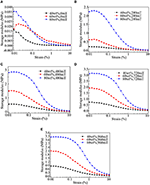Figure 6. Storage modulus of samples as a function of strain under different magnetic flux densities. (A: B = 0mT; B: B = 240mT; C: B = 480mT; E: B = 720mT; E: B = 960mT).

As shown in Figure 6A, in the absence of an external magnetic flux density, storage modulus decreases rapidly along shear strain amplitude and then tends to stabilize beyond certain strain. It can be concluded that the ability of energy storage for PU matrix decreases with the increase of shear strain amplitude. With the influence of the magnetic flux density, as can be seen in Figures 6B–E, MR gels exhibit obvious linear viscoelastic region at the small strain range, which was the same as the dynamic properties of MR grease (Wang et al., 2019). It should be noted that the data is plotted with logarithmic horizontal axis to clear present the linear region. The shear strain amplitude has great impact on the storage modulus of samples. Firstly, the storage modulus of the material does not change with the increase of strain amplitude at small shear strain, indicating the linear viscoelasticity (LVE). Further increasing with the strain amplitude, the storage modulus decreases with the strain amplitude, which is called Payne effect (Arief and Mukhopadhyay, 2015), i.e., the impact of the applied strain amplitude on the storage modulus. Above an approximately 0.1% strain, the storage modulus decrease rapidly along with applied strain amplitude. This threshold strain is called the critical strain amplitude of the material and is dependent on the fillers of the content in the matrix. The critical strain increases along with magnetic flux density and mass fraction of the CIP content for MRG and the critical strain values of samples under different magnetic flux density is shown in Table 2.

TABLE 2

The linear viscoelastic properties of the MR gels have special physical meaning: in the linear viscoelastic region, the internal structure of the material is elastic deformation under shear strain. Once withdrawn, the structure is restored to its original shape, and the change has a continuous and reversible property.

Another important measure of the MR material is the MR effect. The MR effect is related to the zero field storage modulus and magneto-induced storage modulus at the same time. It increases with the increase of magneto-induced storage modulus and decreases with the increase of zero-field modulus. The specific expressions are as follows (Xu et al. 2011):

where is storage modulus under magnetic field, is the storage modulus without applied magnetic field, is magneto-induced storage modulus.

The magnetic flux density dependence of storage modulus for the materials is shown in Figure 7. It is found that the storage moduli of materials increase with the increase of CIP content under the same magnetic flux density. Interestingly, the MR effect of the 60 wt% MR gel is higher than that of 80 wt% MR gel (as shown in Figure 8). The MR effect of the samples with different mass concentrations of CIPs under different magnetic flux densities are shown in Figure 8. It should be noted that the MR effect was calculated based on the storage moduli measured at maximum strain value of linear viscoelastic range at saturated magnetic field flux density 960 mT and zero magnetic field. As indicated in Figure 6 and Table 2, the strain value used for MR effect calculation is 0.02% below which is the strain to define linear viscoelastic range of the MR gel for all magnetic field levels. Each measurement was repeated five times to ensure accuracy of data and then the average values and standard error were taken. Among three samples, the 60 wt% MR gel has demonstrated the highest MR effect. In addition, it was shown that the MR effect does not increase along with the CIPs content. For instance, for MRG-60 and MRG-80, the MR effect at the magnetic flux density of 240, 480, 720, and 960 mT decreases as shown in Figure 8. Such behavior attributes to the off-state modulus of the samples, with MRG-80 has highest off-state modulus (Xu et al., 2011). The MR effect is determined by two factors in the LVE region, which are (i) the induced storage modulus of MR samples under magnetic field due to inter-action between the CIP particles, and (ii) the off-state storage modulus of the samples. Higher CIP content may increase the formation of the chain structure in the sample and lead to higher storage modulus. However, the high off-state shear modulus due to the high particle concentration compromises the relative increase of the modulus.

FIGURE 7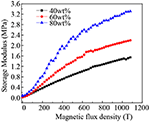Figure 7. The magnetic flux density dependence of storage modulus for the samples with different mass concentration of CIPs.

FIGURE 8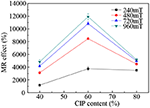Figure 8. The MR effect of the samples with different mass concentration of CIPs under different magnetic flux density.

The storage properties and loss behavior of the samples were studied by scanning strain frequency from 5 to 100 Hz under different magnetic field at shear strain amplitude of 0.01%, which is within the linear viscoelastic range for all samples. The results are shown in Figures 9, 10, respectively.

FIGURE 9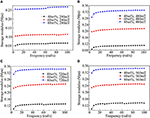Figure 9. Storage modulus as a function of angular frequency. (A: B = 240mT; B: B = 480mT; C: B = 720mT; D: B = 960mT).

FIGURE 10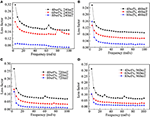Figure 10. Loss factor as a function of angular frequency. (A: B = 240mT; B: B = 480mT; C: B = 720mT; D: B = 960mT).

Figure 9 shows the characteristic curves of storage modulus-frequency of the samples under different magnetic flux densities. At low frequency (<10 Hz), the shear frequency has a great influence on the storage modulus and increases with the increase of the storage modulus. However, the frequency dependence of storage modulus disappears when the frequency is beyond 10 Hz. This interesting phenomenon may be caused by changes in the internal microstructures of PU-based MR gels: PU-based matrix polymers in MR gels intertwined with each other initially. The orientation of these polymer chains distributes along the shear direction under the action of shear oscillation (Zhang et al., 2018). Therefore, the resistance of particles moving in the matrix decreases under the application of magnetic flux density, which makes the storage modulus of MR gels increase firstly. This phenomenon is more obvious at the higher magnetic flux density. However, further increasing the shear frequency, the storage modulus no long changes, which indicates that the weakening of the storage modulus caused by molecular chain winding is compensated under low shear frequency. From Figure 9, in addition, it was shown that elastic performance (storage modulus) is also strongly dependent on CIPs mass fraction, which increases along CIPs mass fraction under the magnetic field.

The loss factor is an important parameter that was determined by both the storage and loss modulus of materials. The specific influence mechanism is explained by the following definition: the loss factor can be defined as the ratio of loss modulus to storage modulus. Therefore, the loss factor characterizes the damping performance of materials (Chandra et al., 1999). When the frequency is <20 Hz, loss factor of samples shows a downward trend along with strain frequency. However, when the frequency is higher than 20 Hz, the frequency dependence of loss factor nearly disappears for both samples under the magnetic flux density. As we all know, the loss factor has something to do with the ability of energy dissipation for the material. This is mainly due to the interaction of different structures within the materials. That is to say the energy dissipation of as-prepared MR gels primarily determined by the interaction between CIPs and PU-based matrix. The position of CIPs changes under low frequency shear. During this process, the movement of CIPs are hindered by PU-based polymer chains and energy dissipation occurs. However, when the frequency increases to the threshold, the segment with strong partial bound has not yet been cut off, so the loss factor decreases along with applied shear frequency and finally tends to a stable state.

## Conclusions

Three PU-based MRGs with a CIPs mass fraction of 40, 60, and 80% were prepared and named MRG-40, MRG-60, and MRG-80, respectively. The static and dynamic tests of the materials were carried out. The conclusions are as follows: the influence of magnetic flux density on the mechanical properties of samples is remarkable, and its tendency with magnetic flux density can be divided into the initial growth, the linear growth and the saturate tendency. In the absence of an external magnetic flux density, the shear stress increases linearly with the increase of the shear rate, which showing Newtonian fluid characteristics. The shear stress of the samples increases with the increment of the CIPs percentage. The shear strain amplitude has different influence on the storage modulus of the materials under different magnetic flux density, and the amplitude of LVE critical strain increases with the increase of magnetic flux density and CIPs mass fraction. It was found that the relative MR effect does not increase with the increasing of CIPs content. Storage modulus is also strongly dependent on CIPs mass fraction, which increases along CIPs mass fraction under the magnetic flux density while loss factor has the opposite trend.

## Author Contributions

GZ conducted the research and wrote the paper. HW assisted the research. YL and JW supervised the research. YL edited the paper.

## Funding

This work is supported by the National Natural Science Foundation of China (No.51675280, No.51805209, and No.51705467) and the Postgraduate Scientific Innovation Research Foundation of Jiangsu Province (NO. KYCX18_0457).

## Conflict of Interest Statement

The authors declare that the research was conducted in the absence of any commercial or financial relationships that could be construed as a potential conflict of interest.

## References

An, H. N., Picken, S. J., and Mendes, E. (2012). Direct observation of particle rearrangement during cyclic stress hardening of magnetorheological gels. Soft Matter 8, 11995–12001. doi: 10.1039/c2sm26587

Arief, I., and Mukhopadhyay, P. K. (2015). Magnetorheological Payne effect in bidisperse MR fluids containing Fe nanorods and Fe3O4, nanospheres: a dynamic rheological study. J. Alloys Comp. 696, 1053–1058. doi: 10.1016/j.jallcom.2016.12.039

Chandra, R., Singh, S. P., and Gupta, K. (1999). Damping studies in fiber-reinforced composites-a review. Compos. Struct. 46, 41–51. doi: 10.1016/S0263-8223(99)00041-0

Fuchs, A., Xin, M., Gordaninejad, F., Wang, X., Hitchcook, G. H., Gecol, H., et al. (2004). Development and characterization of hydrocarbon polyol polyurethane and silicone magnetorheological polymeric gels. J. Appl. Polym. Sci. 92, 1176–1182. doi: 10.1002/app.13434

Gan, J., He, X., Zhang, G., Zhou, C., Zheng, Q., Gao, C., et al. (2017). Study on off-state characteristics of polyurethane based magnetorheological gels. Mater. Sci. Eng. R. 231:012168. doi: 10.1088/1757-899X/231/1/012168

Hu, B., Fuchs, A., Huseyin, S., Gordaninejad, F., and Evrensel, C. (2010). Supramolecular magnetorheological polymer gels. J. Appl. Polym. Sci. 100, 2464–2479. doi: 10.1002/app.23578

Ju, B., Yu, M., Fu, J., Zheng, X., and Liu, S. (2013). Magnetic field-dependent normal force of magnetorheological gel. Ind. Eng. Chem. Res. 52, 11583–11589. doi: 10.1021/ie4013419

Miao, Y., Ju, B., Jie, F., Liu, S., and Choi, S. B. (2014). Magnetoresistance characteristics of magnetorheological gel under a magnetic field. Ind. Eng. Chem. Res. 53, 4704–4710. doi: 10.1021/ie4040237

Mitsumata, T., and Abe, N. (2011). Giant and reversible magnetorheology of carrageenan/iron oxide magnetic gels. Smart Mater. Struct. 20:124003. doi: 10.1088/0964-1726/20/12/124003

Mordina, B., Tiwari, R. K., Setua, D. K., and Sharma, A. (2016). Impact of graphene oxide on the magnetorheological behaviour of BaFe12O19, nanoparticles filled polyacrylamide hydrogel. Polymer 97, 258–272. doi: 10.1016/j.polymer.2016.05.026

Shiga, T., Okada, A., and Kurauchi, T. (1995). Magnetroviscoelastic behavior of composite gels. J. Appl. Polym. Sci. 58, 787–792. doi: 10.1002/app.1995.070580411

Wang, H., Li, Y., Zhang, G., and Wang, J. (2019). Effect of temperature on rheological properties of lithium-based magnetorheological grease. Smart Mater. Struct. 28:035002. doi: 10.1088/1361-665X/aaf32b

Wang, Y., Wang, S., Xu, C., Xuan, S., Jiang, W., Gong, X., et al. (2016). Dynamic behavior of magnetically responsive shear-stiffening gel under high strain rate. Compos. Sci. Technol. 127, 169–176. doi: 10.1016/j.compscitech.2016.03.009

Wei, B., Gong, X., Jiang, W., Qin, L., and Fan, Y. (2010). Study on the properties of magnetorheological gel based on polyurethane. J. Appl. Polym. Sci. 118, 2765–2771. doi: 10.1002/app.32688

Wilson, M. J., Fuchs, A., and Gordaninejad, F. (2002). Characterization of magnetorheological polymer gels. J. Appl. Polym. Sci. 84, 2733–2742. doi: 10.1002/app.10525

Xu, Y., Gong, X., and Xuan, S. (2013). Soft magnetorheological polymer gels with controllable rheological properties. Smart Mater. Struct. 22:075029. doi: 10.1088/0964-1726/22/7/075029

Xu, Y., Gong, X., Xuan, S., Zhang, W., and Fan, Y. (2011). A high-performance magnetorheological material: preparation, characterization and magnetic-mechanic coupling properties. Soft Matter 7, 5246–5254. doi: 10.1039/c1sm05301a

Xu, Y., Liu, T., Liao, G. J., and Lubineau, G. (2017). Magneto-dependent stress relaxation of magnetorheological gels. Smart Mater. Struct. 26:115005. doi: 10.1088/1361-665X/aa89ac

Yang, P., Yu, M., Fu, J., Liu, S., Qi, S., and Zhu, M. (2015). The damping behavior of magnetorheological gel based on polyurethane matrix. Polym. Compos. 38, 1248–1258. doi: 10.1002/pc.23689

Zhang, G., Wang, H. X., Ouyang, Q., Wang, J., and Zhen, J. (2018). Study on the rheological properties of magnetorheological gel based on silicone and Herschel-Bulkley model parameter identification. J. Hunan Univ. 45, 62–71. doi: 10.16339/j.cnki.hdxbzkb.2018.06.010

CrossRef Full Text

Zhang, W., Gong, X., Xuan, S., and Jiang, W. (2011). Temperature-dependent mechanical properties and model of magnetorheological elastomers. Ind. Eng. Chem. Res. 50, 6704–6712. doi: 10.1021/ie200386x

Zhang, W., Gong, X. L., Xuan, S. H., and Xu, Y. (2010). High-performance hybrid magnetorheological materials: preparation and mechanical properties. Ind. Eng. Chem. Res. 49, 12471–12476. doi: 10.1021/ie101904f

Keywords: magnetorheological gel, rotation shear, oscillatory shear, Payne effect, loss factor, linear viscoelasticity

Citation: Zhang G, Li Y, Wang H and Wang J (2019) Rheological Properties of Polyurethane-Based Magnetorheological Gels. Front. Mater. 6:56. doi: 10.3389/fmats.2019.00056

Received: 26 November 2018; Accepted: 20 March 2019;
Published: 10 April 2019.

Edited by:

Miao Yu, Chongqing University, China

Reviewed by:

Luwei Zhou, Fudan University, China
Xianzhou Zhang, Independent Researcher, Tomago, NSW, Australia

Copyright © 2019 Zhang, Li, Wang and Wang. This is an open-access article distributed under the terms of the Creative Commons Attribution License (CC BY). The use, distribution or reproduction in other forums is permitted, provided the original author(s) and the copyright owner(s) are credited and that the original publication in this journal is cited, in accordance with accepted academic practice. No use, distribution or reproduction is permitted which does not comply with these terms.

*Correspondence: Yancheng Li, yli@njtech.edu.cn; yancheng.li@uts.edu.au
Jiong Wang, wjiongz@njust.edu.cn